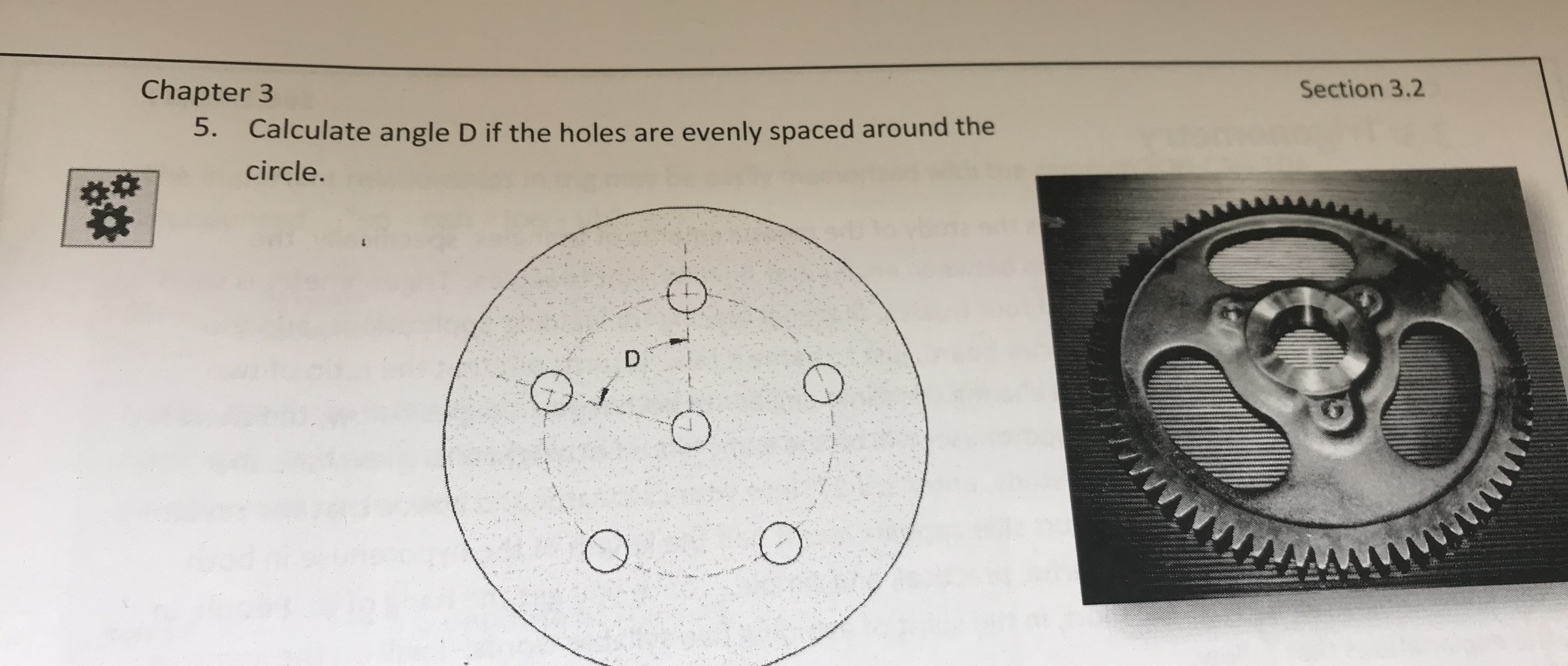Section 3.2Chapter 35.Calculate angle D if the holes are evenly spaced around thecircle

Question

Please provide detailed explanation, easy to understand.help_outlineImage TranscriptioncloseSection 3.2 Chapter 3 5. Calculate angle D if the holes are evenly spaced around the circle fullscreen
Step 1

Given that, the holes are evenly spaced around the circle.

It is observed that five holes are there in the circle.

It is known that, the angle of full circle is 360 degrees.

Step 2

To calculate the angle of D, divide the angle of full circle ...

Want to see the full answer?

See Solution

Want to see this answer and more?

Our solutions are written by experts, many with advanced degrees, and available 24/7

See Solution
Tagged in

Circles